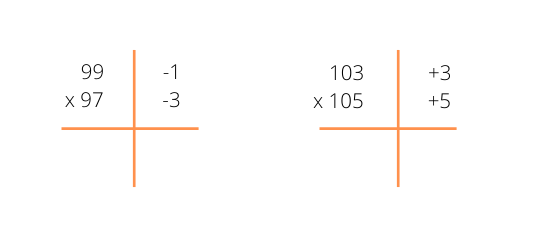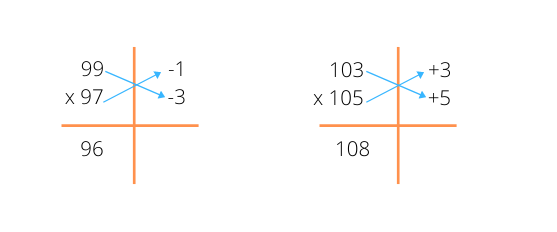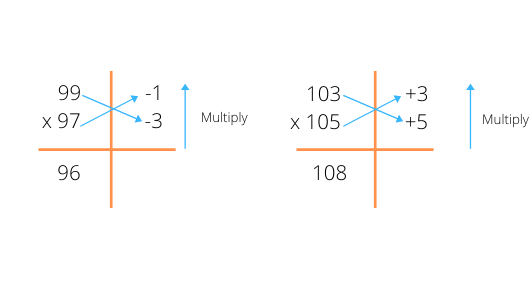# Vedic Maths:- multiply two numbers close to 100 i.e 98, 102, etc

By ac05 | Vedic Maths | 6 May 2021

Let us understand it by taking two examples.

• Multiply 99 × 97

• Multiply 103 × 105

Steps to perform this are:-

• Find by how much the number is more or less than the power of 100 and write it on the right side of the vertical line.• Either cross subtracts or cross add. This will form the first part of the final answer.• Multiply the right side of the vertical line and this shall form the right side of the result.03                                                          15

• Add this multiplied number to the end giving 9603 and 10815 respectively.

This is how you can multiply numbers close to 100 in just 3 steps.

• 102 x 105
• 98 x 93
• 99 x 99

thank you!ac05

A competitive Programmer exploring different domainsVedic Maths

Vedic mathematics consists of various techniques that makes calculations easy and fast. Well i will be posting some of the vedic maths tricks, made by ancient Indian mathematics. If you grasp those tricks you could easily do calculation in your mind. Now you won't need calculator every time to do addition or multiplication or squaring or even square rooting a number. At the end of each post I will be adding few questions that you must do with those tricks without using calculator. Have a nice day!Send a \$0.01 microtip in crypto to the author, and earn yourself as you read!

20% to author / 80% to me.
We pay the tips from our rewards pool.